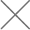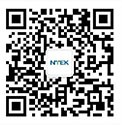mtbf的计算

Ti——第i台被试整机的累计工作时间，h；

rn——被试整机在试验期间内出现的故障总数。

MTBF = Total Test Time*Acceleration Factor/Coefficient (refer to the table)

Total Test Time = (sample size)*(test days)*(power on hour/day)

Acceleration Factor = e (Ea/Kb) (1/Tn-1/Ta)

Ta = Burn – in Room Test Temperature

Tn = Normal Temperature

t=10小时 * 365天 * 4年=14600小時

MTBF= 1/λ=70000

F(14600)=1-e-14600/70000=1-e-0.21=0.1882=18.82%

R(14600)=1-0.19=0.8118=81%

t=10h* 30天* 6个月=1800小時

MTBF= 1/λ=70000

6个月后失效概率:

F(14600)=1-e-1800/70000=1-e-0.0257=0.0254=2.54%

6个月后可靠度:

R(14600)=1-0.0254=0.9746=97.46%

MTBF计算公式（通过温度系数）:

MTBF = Total Test Time*Acceleration Factor/Coefficient (refer to the table)

AF：Accelerate Factor，加速因子

T：Total Power on Time，总的开机运行时间

X2(α,2r+2)：卡方公式

C：Confidential Level，信心度水平

α：生产者的冒险率，即：1-C

r：失效数，Number of Failures

MTBF测试，Burn-in测试，ALT区别:

 - MTBF测试 Burn-in测试 ALT加速寿命测试 测试环境 高温房 高温房 高温房 测试温度 37.5℃±2.5℃ 37.5℃±2.5℃ 37.5℃±2.5℃ 测试时间 长 短 长，但比MTBF短 测试目的 验证产品符合所设计的功能及用户的可靠性要求 剔除不合格或有可能早期失效的产品 采用加大应力的方法促使样品在短期内失效，以观测在正常工作条件或储存条件下的可靠性

Burn-in：“老化”测试，指产品在规定的应力条件下，使其特性达到稳定的方法。

ALT：Accelerated life test，加速寿命测试，是在超过使用环境条件的应力水平下对样品进行的寿命试验。

NTEK北测检测，专业第三方可靠性检测机构，作为国家级高新技术企业、新型材料及电子产品综合公共测试平台，是中国第三方检测认证服务的开拓者，也是国内最早从事商品检验、鉴定、测试、认证等服务的专业机构之一，独立第三检测、测试、认证机构，多种检测设备，资深测试、认证工程师，为客户提供一站式检测、测试、认证服务。

•微信公众号

•微信咨询